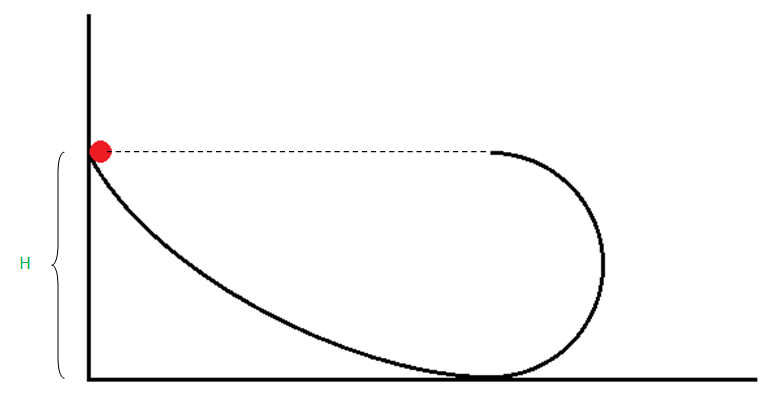# Conserve Energy?A small body starts sliding from the height $H$ down a fixed inclined groove passing into a half circle of radius $\frac{H}{2}$. Find the speed of body (in m/s) when it reaches the highest point (w.r.t the lowest point of circular path) of its trajectory after entering into the half circle.

Given Data : $H = 5.4 m$ & $g = 9.8 ms^{-2}$

Try more from my set Classical Mechanics Problems.

×

Problem Loading...

Note Loading...

Set Loading...